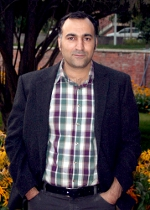Email: esfahani (@) sharif.ir
Phone: +98-21-66165646
Fax: +98-21-66005117
Department of Mathematical Sciences
Sharif University of Technology
P.O. Box: 11155-9415
Tehran, Iran

## Publications

• Heat equation approach to an index theorem on odd dimensional manifolds (Bull. Belg. Math. Soc. Simon Stevin 16 (2009), no. 4, 647-664).

• Morse inequalities for manifolds with boundary (J. Korean Math. Soc. 47 (2010), no. 1, 123–134).

• Index theory and partitioning by enlargeable hyper surfaces (J. Noncommut. Geom. 4 (2010), no. 3, 459–473).

• Delocalized Betti numbers and Morse type inequalities (Rocky Mountain J. Math. 41 (2011), no. 4, 1361–1374).

• A higher index theorem on foliated manifolds with boundary (Bull. Sci. Math. 136 (2012), no. 2, 201–238.).

• A note on some classical results of Gromov-Lawson (Proc. Amer. Math. Soc. 140 (2012), no. 10, 3663–3672)

• On vanishing of low degree higher A-genera (Differential Geometry and its Applications,Volume 43, December 2015, Pages 56-59)

• (joint work with Th. Schick) Large scale index of multi-partitioned manifolds (Journal of Noncommutative Geometry, vol 12, N 2, pp. 439-456)

•  (joint work with M. Karami and A. R.H.S Sadegh) A Coarse Relative-Partitioned index theorem ( Bulletin des sciences mathematiques, volume 153, July 2019, Pages 57-71)

• (joint work with R. Moghadasi) Analytic approach to S¹-equivariant Morse inequalities (Algebraic & Geometric Topology 22 (2022) 3059–3082)

Preprints:

• A Concise course in Index Theory

• On cut-and-past invariance of Kervaire semi-characteristic